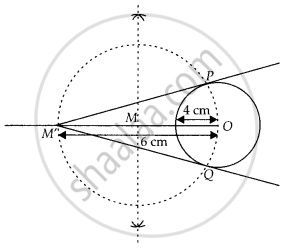# Construct a tangent to a circle of radius 4 cm from a point which is at a distance of 6 cm from its centre. - Mathematics

Diagram
Sum

Construct a tangent to a circle of radius 4 cm from a point which is at a distance of 6 cm from its centre.

#### Solution

Given, a point M’ is at a distance of 6 cm from the centre of a circle of radius 4 cm.

Steps of construction:

1. Draw a circle of radius 4 cm. Let centre of this circle is O.

2. Join OM’ and bisect it. Let M be mid-point of OM’.

3. Taking M as centre and MO as radius draw a circle to intersect circle (0, 4) at two points, P and Q.

4. Join PM’ and QM’. PM’ and QM’ are the required tangents from M’ to circle C(0, 4).Concept: Construction of Tangents to a Circle
Is there an error in this question or solution?

#### APPEARS IN

NCERT Mathematics Exemplar Class 10
Chapter 10 Construction
Exercise 10.3 | Q 4 | Page 116
Share